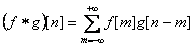# Convolution

The following operation is called a discrete convolution of functions f(t) and g(t) (both functions are defined on Z):The following operation is called a circular discrete convolution of a nonperiodic function f and a periodic function g:A discrete convolution has many various purposes - multiplication of polynomials, arbitrary precision arithmetics and signal processing. Circular convolution is non-commutative: one of the functions is a periodic signal and the other is a non periodic response to the signal. Operands of non-circular convolution often have different context as well, but the operation itself is commutative: the result of convolution does not change if the functions f and g switch places.

# Data representation

The domain of f and g is Z, but in practice we deal with data with restricted length. The most convenient case is when functions f(t) and g(t) are nonzero only for non-negative t. The ALGLIB package solve this problem: a convolution of two functions, which are nonzero only for non-negative values of the argument. This allows to use a simple relation between the function argument and the array index, where its values are kept: f(t=i) = f_array[i], 0 ≤ i < M and g(t=i) = g_array[i], 0 ≤ i < N.

In case the functions f and g are nonzero both for positive and negative values of the argument, one can utilize the fact that when one of the convolution arguments is shifted, the result is also shifted in the same direction by the same value (if both arguments are shifted, the result is shifted by the sum of individual shifts). Just shift f and g to the right until all the nonzero values are on one side of zero, call a convolution subroutine and then shift the result back.

# Convolution implementation in ALGLIB

For simplicity of use let's presume that N ≥ M, i.e. the second operand is longer than the first, even though the speed of the algorithm does not depend on the order in which the operands are given. It is widely known that a convolution can be calculated using fast Fourier transform in time O(N·log(N)). However, the straightforward usage of FFT is not always the optimal solution: in case one of the operands is shorter than the other, one can seriously speed up the calculations by using other algorithms. Depending on the operand length, ALGLIB package can use the following algorithms:

• If M is not large, an algorithm with O(M·N) complexity.
• If M is substantially smaller than N, but still too large to use the first algorithm, an overlap-add algorithm with O(N·log(M)) complexity is used.
• If two previous algorithms don't speed up the process, the package uses an algorithm based on FFT with O(N·log(N)) complexity (first, the operands undergo FFT, then components of frequencies are multiplied and then there is a inverse FFT). The convolution subroutine automatically selects the length of the operands adding zeros when necessary to achieve optimal speed (the speed of FFT strongly depends on factorization of its length). Thus, ALGLIB users don't have to worry about the optimal operand length.

ALGLIB Project offers you two editions of ALGLIB:

ALGLIB Free Edition:delivered for freeoffers full set of numerical functionalityextensive algorithmic optimizationsno low level optimizationsnon-commercial license

ALGLIB Commercial Edition:flexible pricingoffers full set of numerical functionalityextensive algorithmic optimizationshigh performance (SMP, SIMD)commercial license with support plan

## ALGLIB 3.16.0 for C++C++ library.
Delivered with sources.
Monolithic design.
Extreme portability.
Editions:   FREE   COMMERCIAL

## ALGLIB 3.16.0 for C#C# library with native kernels.
Delivered with sources.
VB.NET and IronPython wrappers.
Extreme portability.
Editions:   FREE   COMMERCIAL

## ALGLIB 3.16.0 for DelphiDelphi wrapper around C core.
Delivered as precompiled binary.
Compatible with FreePascal.
Editions:   FREE   COMMERCIAL

## ALGLIB 3.16.0 for CPythonCPython wrapper around C core.
Delivered as precompiled binary.
Editions:   FREE   COMMERCIAL